## Suppose you observed the equation for a traveling wave to be y(x, t) = A cos(kx − ????t), where its amplitude of oscillations was 0.15 m, it

Question

Suppose you observed the equation for a traveling wave to be y(x, t) = A cos(kx − ????t), where its amplitude of oscillations was 0.15 m, its wavelength was two meters, and the period was 2/15 s. If a point on the wave at a specific time has a displacement of 0.12 m, what is the transverse speed of that point?

in progress 0
6 months 2021-08-10T15:27:02+00:00 1 Answers 2 views 0

## 15m/s

Explanation:

The equation for a traveling wave as expressed as y(x, t) = A cos(kx −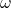t) where An is the amplitude f oscillation,is the angular velocity and x is the horizontal displacement and y is the vertical displacement.

From the formula;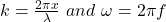where;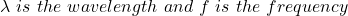Before we can get the transverse speed, we need to get the frequency and the wavelength.

frequency = 1/period

Given period = 2/15 s

Frequency =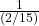frequency = 1 * 15/2

frequency f = 15/2 Hertz

Given wavelength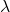= 2m

Transverse speed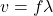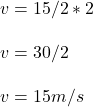Hence, the transverse speed at that point is  15m/s